SBOS424C March   2008  – November 2015

PRODUCTION DATA.

1. Features
2. Applications
3. Description
4. Revision History
5. Device Comparison
6. Pin Configuration and Functions
7. Specifications
8. Detailed Description
9. Applications and Implementation
1. 9.1 Application Information
2. 9.2 Typical Applications
10. 10Power Supply Recommendations
11. 11Layout
1. 11.1 Layout Guidelines
2. 11.2 Layout Example
12. 12Device and Documentation Support
13. 13Mechanical, Packaging, and Orderable Information

Package Options

Refer to the PDF data sheet for device specific package drawings

• PW|20
• PW|20

7 Specifications

7.1 Absolute Maximum Ratings

Over operating free-air temperature range, unless otherwise noted.(1)
MIN MAX UNIT
Supply voltage 7 V
Signal input terminals, voltage(2) GND – 0.5 AVDD + 0.5 V
Signal input terminals, current(2) ±10 mA
Output short circuit Continuous
Operating temperature –40 125 °C
Junction temperature 150 °C
Storage temperature –65 150 °C
(1) Stresses above these ratings may cause permanent damage. Exposure to absolute maximum conditions for extended periods may degrade device reliability. These are stress ratings only, and functional operation of the device at these or any other conditions beyond those specified is not implied.
(2) Input terminals are diode-clamped to the power-supply rails. Input signals that can swing more than 0.5 V beyond the supply rails should be current-limited to 10 mA or less.

7.2 ESD Ratings

VALUE UNIT
V(ESD) Electrostatic discharge Human-body model (HBM), per ANSI/ESDA/JEDEC JS-001(1) ±3000 V
Charged-device model (CDM), per JEDEC specification JESD22-C101(2) ±1000
Machine Model (MM) ±300
(1) JEDEC document JEP155 states that 500-V HBM allows safe manufacturing with a standard ESD control process.
(2) JEDEC document JEP157 states that 250-V CDM allows safe manufacturing with a standard ESD control process.

7.3 Recommended Operating Conditions

over operating free-air temperature range (unless otherwise noted)
MIN NOM MAX UNIT
AVDD 2.2 5 5.5 V
DVDD 2.2 5 5.5 V
Operating temperature –40 25 125 °C

7.4 Thermal Information

THERMAL METRIC(1) PGA112, PGA113 PGA116, PGA117 UNIT
DGS (VSSOP) PW (TSSOP)
10 PINS 20 PINS
RθJA Junction-to-ambient thermal resistance 98.3 100.3 °C/W
RθJC(top) Junction-to-case (top) thermal resistance 57 36.9 °C/W
RθJB Junction-to-board thermal resistance 51.2 50.6 °C/W
ψJT Junction-to-top characterization parameter 1.3 2.6 °C/W
ψJB Junction-to-board characterization parameter 36.9 50.2 °C/W
RθJC(bot) Junction-to-case (bottom) thermal resistance 4.8 N/A °C/W

7.5 Electrical Characteristics: VS = AVDD = DVDD = 5 V

at TA = 25°C, RL = 10kΩ//CL = 100 pF connected to DVDD/2, and VREF = GND (unless otherwise noted)
PARAMETER TEST CONDITIONS MIN TYP MAX UNIT
OFFSET VOLTAGE
Input offset voltage VOS AVDD = DVDD = 5 V, VREF = VIN = AVDD/2, VCM = 2.5 V ±25 ±100 μV
AVDD = DVDD = 5 V, VREF = VIN = AVDD/2, VCM = 4.5 V ±75 ±325 μV
vs temperature, –40°C to 125°C dVOS/dT AVDD = DVDD = 5 V, VCM = 2.5 V TA = –40°C to 125°C 0.35 1.2 μV/°C
vs temperature, –40°C to 85°C AVDD = DVDD = 5 V, VCM = 2.5 V 0.15 0.9 μV/°C
vs temperature, –40°C to 125°C AVDD = DVDD = 5 V, VCM = 4.5 V TA = –40°C to 125°C 0.6 1.8 μV/°C
vs temperature, –40°C to 85°C AVDD = DVDD = 5 V, VCM = 4.5 V 0.3 1.3 μV/°C
vs power supply PSRR AVDD = DVDD = 2.2 V to 5.5 V, VCM = 0.5 V,
VREF = VIN = AVDD/2
5 20 μV/V
Over temperature, –40°C to 125°C AVDD = DVDD = 2.2 V to 5.5 V, VCM = 0.5 V,
VREF = VIN = AVDD/2
TA = –40°C to 125°C 5 40 μV/V
INPUT ON-CHANNEL CURRENT
Input on-channel current (Ch0, Ch1) IIN VREF = VIN = AVDD/2 ±1.5 ±5 nA
Over temperature, –40°C to 125°C VREF = VIN = AVDD/2 See Typical Characteristics nA
INPUT VOLTAGE RANGE
Input voltage range(1) IVR GND – 0.1 AVDD + 0.1 V
Overvoltage input range No output phase reversal(2) GND – 0.3 AVDD + 0.3 V
INPUT IMPEDANCE (Channel On)(3)
Channel input capacitance CCH 2 pF
Channel switch resistance RSW 150 Ω
Amplifier input capacitance CAMP 3 pF
Amplifier input resistance RAMP Input resistance to GND 10
VCAL/CH0 RIN CAL1 or CAL2 selected 100
GAIN SELECTIONS
Nominal gains Binary gains: 1, 2, 4, 8, 16, 32, 64, 128 1 128
Scope gains: 1, 2, 5, 10, 20, 50, 100, 200 1 200
DC gain error G = 1 VOUT = GND + 85 mV to DVDD – 85 mV 0.006% 0.1%
1 < G ≤ 32 VOUT = GND + 85 mV to DVDD – 85 mV 0.1%
G ≥ 50 VOUT = GND + 85 mV to DVDD – 85 mV 0.3%
DC gain drift G = 1 VOUT = GND + 85 mV to DVDD – 85 mV TA = –40°C to 125°C 0.5 ppm/°C
1 < G ≤ 32 VOUT = GND + 85 mV to DVDD – 85 mV TA = –40°C to 125°C 2 ppm/°C
G ≥ 50 VOUT = GND + 85 mV to DVDD – 85 mV TA = –40°C to 125°C 6 ppm/°C
CAL2 DC gain error(4) Op Amp + Input = 0.9 VCAL,
VREF = VCAL = AVDD/2, G = 1
0.02%
CAL2 DC gain drift(4) Op Amp + Input = 0.9 VCAL,
VREF = VCAL = AVDD/2, G = 1
TA = –40°C to 125°C 2 ppm/°C
CAL3 DC gain error(4) Op Amp + Input = 0.1 VCAL,
VREF = VCAL = AVDD/2, G = 1
0.02%
CAL3 DC gain drift(4) Op Amp + Input = 0.1 VCAL,
VREF = VCAL = AVDD/2, G = 1
TA = –40°C to 125°C 2 ppm/°C
INPUT IMPEDANCE (CHANNEL OFF)(3)
Input impedance CCH See Figure 55 2 pF
INPUT OFF-CHANNEL CURRENT
Input Off-Channel Current (Ch0, Ch1)(5) ILKG VREF = GND, VOFF-CHANNEL = AVDD/2,
VON-CHANNEL = AVDD/2 – 0.1 V
±0.05 ±1 nA
Over temperature, –40°C to 125°C VREF = GND, VOFF-CHANNEL = AVDD/2,
VON-CHANNEL = AVDD/2 – 0.1 V
See Typical Characteristics
Channel-to-Channel Crosstalk 130 dB
OUTPUT
Voltage output swing from rail IOUT = ±0.25 mA, AVDD ≥ DVDD(7) GND + 0.05 DVDD – 0.05 V
IOUT = ±5 mA, AVDD ≥ DVDD(7) GND + 0.25 DVDD – 0.25 V
DC output nonlinearity VOUT = GND + 85 mV to DVDD – 85 mV(6) 0.0015 %FSR
Short circuit current ISC –30/+60 mA
NOISE
Input voltage noise density en f > 10 kHz, CL = 100 pF, VS = 5 V 12 nV/√Hz
f > 10 kHz, CL = 100 pF, VS = 2.2 V 22 nV/√Hz
Input voltage noise en f = 0.1 Hz to 10 Hz, CL = 100 pF, VS = 5 V 0.362 μVPP
f = 0.1 Hz to 10 Hz, CL = 100 pF, VS = 2.2 V 0.736 μVPP
Input current density In f = 10 kHz, CL = 100 pF 400 fA/√Hz
SLEW RATE
Slew rate SR See Table 1 V/μs
SETTLING TIME
Settling time tS See Table 1 μs
FREQUENCY RESPONSE
Frequency response See Table 1 MHz
THD + NOISE
G = 1, f = 1 kHz, VOUT = 4 VPP at 2.5VDC, CL = 100 pF 0.003%
G = 10, f = 1 kHz, VOUT = 4 VPP at 2.5VDC, CL = 100 pF 0.005%
G = 50, f = 1 kHz, VOUT = 4 VPP at 2.5VDC, CL = 100 pF 0.03%
G = 128, f = 1 kHz, VOUT = 4 VPP at 2.5VDC, CL = 100 pF 0.08%
G = 200, f = 1 kHz, VOUT = 4 VPP at 2.5VDC, CL = 100 pF 0.1%
G = 1, f = 20 kHz, VOUT = 4 VPP at 2.5VDC, CL = 100 pF 0.02%
G = 10, f = 20 kHz, VOUT = 4 VPP at 2.5VDC, CL = 100 pF 0.01%
G = 50, f = 20 kHz, VOUT = 4 VPP at 2.5VDC, CL = 100 pF 0.03%
G = 128, f = 20 kHz, VOUT = 4 VPP at 2.5VDC, CL = 100 pF 0.08%
G = 200, f = 20 kHz, VOUT = 4 VPP at 2.5VDC, CL = 100 pF 0.11%
POWER SUPPLY
Operating voltage range(7) AVDD 2.2 5.5 V
DVDD 2.2 5.5 V
Quiescent current analog IQA IO = 0, G = 1, VOUT = VREF 0.33 0.45 mA
Over temperature, –40°C to 125°C TA = –40°C to 125°C 0.45 mA
Quiescent current digital(8)(9)(10) IQD IO = 0, G = 1, VOUT = VREF, SCLK at 10 MHz,
CS = Logic 0, DIO or DIN = Logic 0
0.75 1.2 mA
Over temperature, –40°C to 125°C(8)(9)(10) IO = 0, G = 1, VOUT = VREF, SCLK at 10 MHz,
CS = Logic 0, DIO or DIN = Logic 0
TA = –40°C to 125°C 1.2 mA
Shutdown current analog + digital(8)(9) ISDA + ISDD IO = 0, VOUT = VREF, G = 1, SCLK Idle 4 μA
IO = 0, VOUT = 0, G = 1, SCLK at 10MHz,
CS = Logic 0, DIO or DIN = Logic 0
245 μA
POWER-ON RESET (POR)
POR trip voltage Digital interface disabled and Command Register set to POR values for DVDD  < POR Trip Voltage 1.6 V
TEMPERATURE RANGE
Specified range –40 125 °C
Operating range –40 125 °C
Thermal resistance θJA
VSSOP-10 164 °C/W
DIGITAL INPUTS (SCLK, CS, DIO, DIN)
Logic low 0 0.3DVDD V
Input leakage current (SCLK and CS only) –1 1 μA
Weak pulldown current (DIO, DIN only) 10 μA
Logic high 0.7DVDD DVDD V
Hysteresis 700 mV
DIGITAL OUTPUT (DIO, DOUT)
Logic high IOH = –3 mA (sourcing) DVDD – 0.4 DVDD V
Logic low IOL = 3 mA (sinking) GND GND + 0.4 V
CHANNEL AND GAIN TIMING
Channel select time 0.2 μs
Gain select time 0.2 μs
SHUTDOWN MODE TIMING
Enable time 4 μs
Disable time VOUT goes high-impedance, RF and RI remain connected between VOUT and VREF 2 μs
POWER-ON-RESET (POR) TIMING
POR power-up time DVDD ≥ 2 V 40 μs
POR power-down time DVDD ≤ 1.5 V 5 μs
(1) Gain error is a function of the input voltage. Gain error outside of the range (GND + 85 mV ≤ VOUT ≤ DVDD – 85 mV) increases to 0.5% (typical).
(2) Input voltages beyond this range must be current-limited to < |10 mA| through the input protection diodes on each channel to prevent permanent destruction of the device.
(3) See Figure 55.
(4) Total VOUT error must be computed using input offset voltage error multiplied by gain. Includes op amp G = 1 error.
(5) Maximum specification limitation limited by final test time and capability.
(6) Measurement limited by noise in test equipment and test time.
(7) When AVDD is less than DVDD, the output is clamped to AVDD + 300 mV.
(8) Does not include current into or out of the VREF pin. Internal RF and RI are always connected between VOUT and VREF.
(9) Digital logic levels: DIO or DIN = logic 0. 10-μA internal pulldown current source.
(10) Includes current from op amp output structure.

7.6 SPI Timing: VS = AVDD = DVDD = 2.2 V to 5 V

At TA = +25°C, RL = 10kΩ//CL = 100pF connected to DVDD/2, and VREF = GND, unless otherwise noted.
MIN NOM MAX UNIT
Input capacitance (SCLK, CS, and DIO pins) 1 pF
tRFI Input rise and fall time(1)
(CS, SCLK, and DIO pins)
2 μs
tRFO Output rise and fall time (DIO pin)(1) CLOAD = 60 pF 10 ns
tCSH CS high time (CS pin)(1) 40 ns
tCSO SCLK edge to CS fall setup time(1) 10 ns
tCSSC CS fall to first SCLK edge setup time 10 ns
fSCLK SCLK Frequency(2) 10 MHz
tHI SCLK high time(3) 40 ns
tLO SCLK low time(3) 40 ns
tSCCS SCLK last edge to CS rise setup time(1) 10 ns
tCS1 CS rise to SCLK edge setup time(1) 10 ns
tSU DIN setup time 10 ns
tHD DIN hold time 10 ns
tDO SCLK to DOUT valid propagation delay(1) 25 ns
tSOZ CS rise to DOUT forced to Hi-Z(1) 20 ns
(1) Ensured by design; not production tested.
(2) When using devices in daisy-chain mode, the maximum clock frequency for SCLK is limited by SCLK rise and fall time, DIN setup time, and DOUT propagation delay. See Figure 61. Based on this limitation, the maximum SCLK frequency for daisy-chain mode is 9.09 MHz.
(3) tHI and tLO must not be less than 1/SCLK (maximum).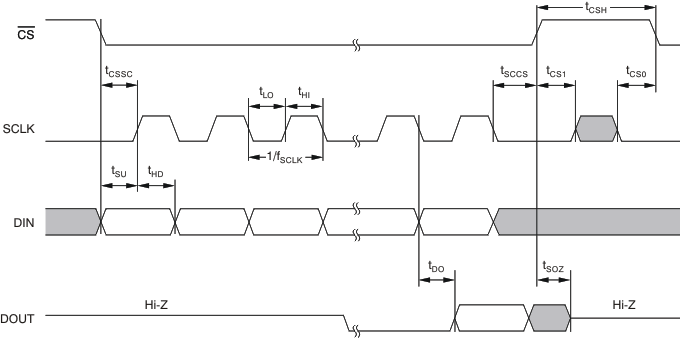Figure 1. SPI Mode 0, 0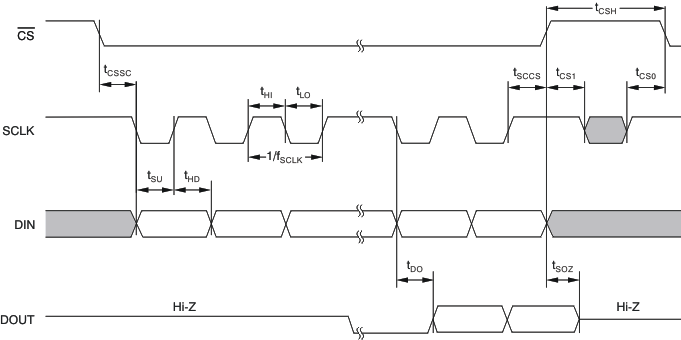Figure 2. SPI Mode 1, 1

7.7 Typical Characteristics

at TA = 25°C, AVDD = DVDD = 5 V, RL = 10 kΩ connected to DVDD/2, VREF = GND, and CL = 100 pF, unless otherwise noted.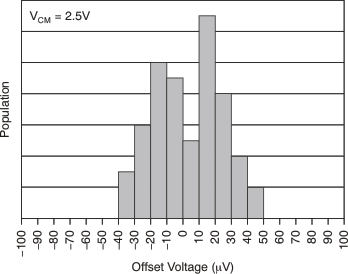Figure 3. Offset Voltage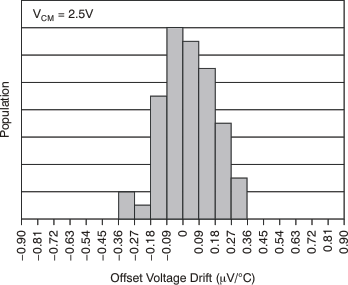Figure 5. Offset Voltage Drift (–40°C to 85°C)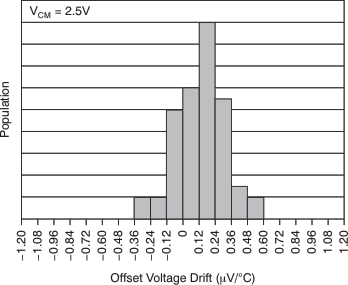Figure 7. Offset Voltage Drift (–40°C to 125°C)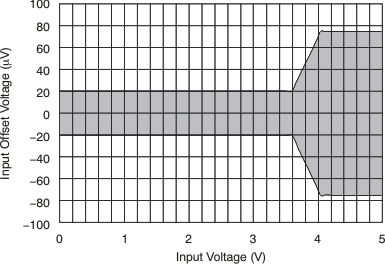Figure 9. Input Offset Voltage vs Input Voltage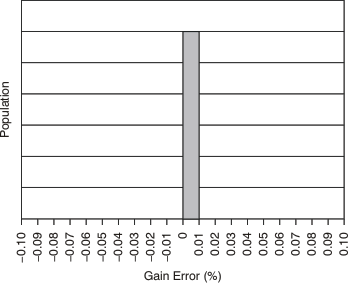Figure 11. Gain Error (G = 1)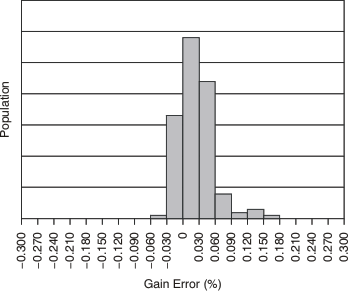Figure 13. Gain Error (G ≥ 50)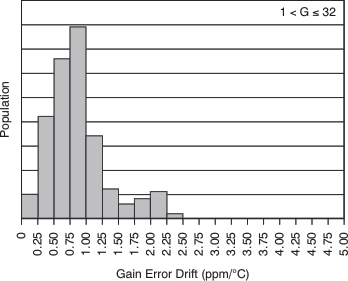Figure 15. Gain Error Drift (–40°C to 125°C)Figure 17. CAL2 Gain Error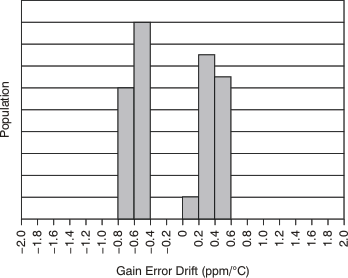Figure 19. CAL2 Gain Error Drift (–40°C to 125°C)Figure 21. 0.1 Hz To 10 Hz NOISE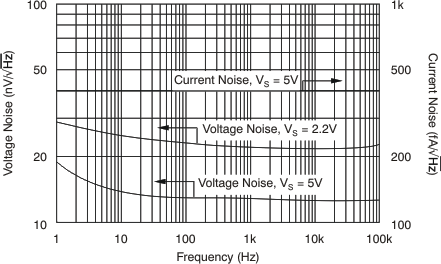Figure 23. Spectral NOISE Density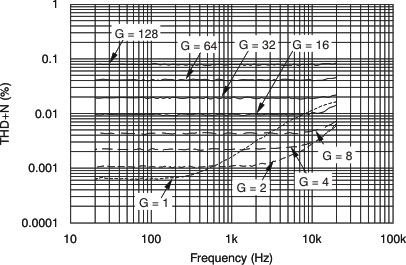Figure 25. PGA112, PGA116 THD + NOISE vs Frequency (VOUT = 4 VPP)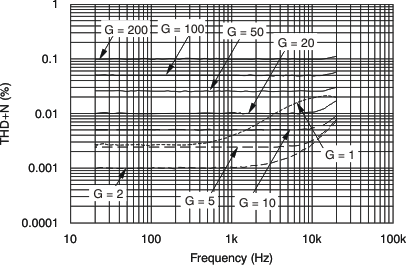Figure 27. PGA113, PGA117 THD + Noise vs Frequency (VOUT = 4 VPP)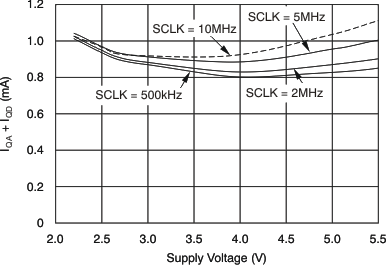Figure 29. Total Quiescent Current vs Supply Voltage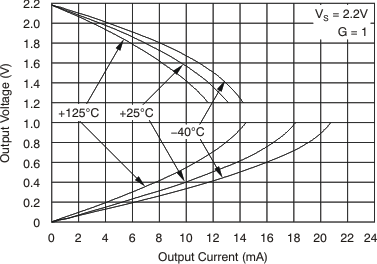Figure 31. Output Voltage vs Output Current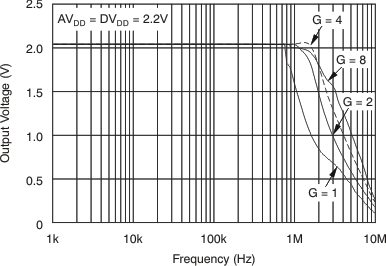Figure 33. PGA112, PGA116 Output Voltage Swing vs Frequency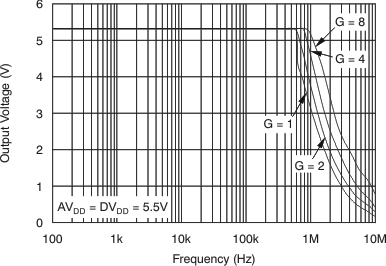Figure 35. PGA112, PGA116 Output Voltage Swing vs Frequency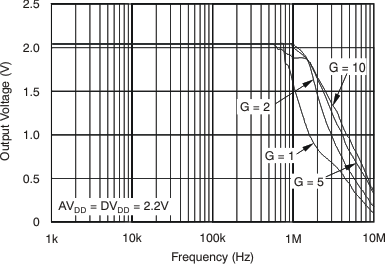Figure 37. PGA113, PGA117 Output Voltage Swing vs Frequency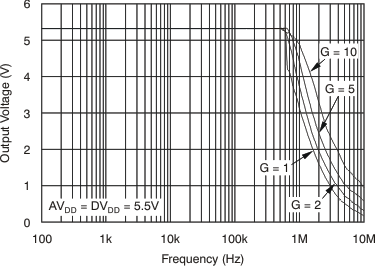Figure 39. PGA113, PGA117 Output Voltage Swing vs Frequency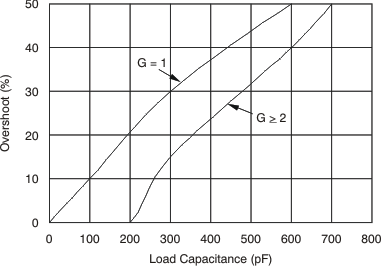Figure 41. Small-Signal Overshoot vs Load CapacitanceFigure 43. Input ON-Channel Current vs Temperature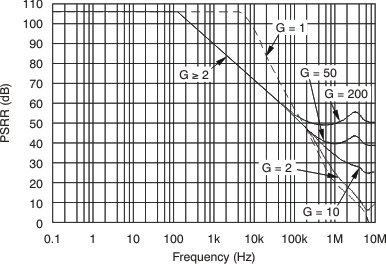Figure 45. Power-Supply Rejection Ratio vs Frequency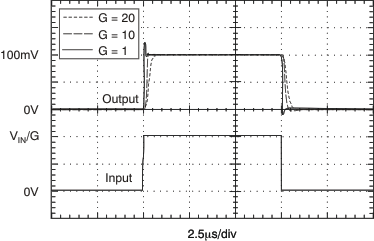Figure 47. Small-Signal Pulse Response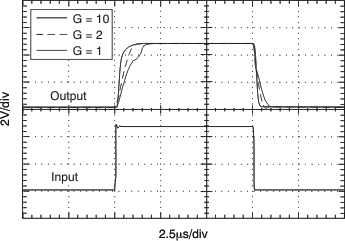Figure 49. Large-Signal Pulse Response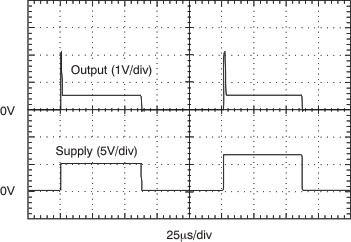Figure 51. Power-Up and Power-Down Timing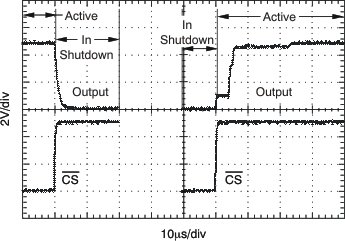Figure 53. Output Voltage vs Shutdown ModeFigure 4. Offset Voltage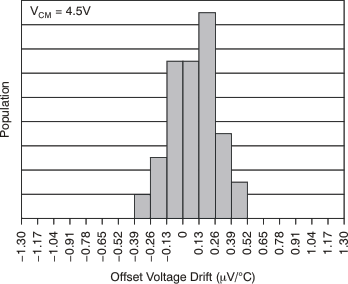Figure 6. Offset Voltage Drift (–40°C to 85°C)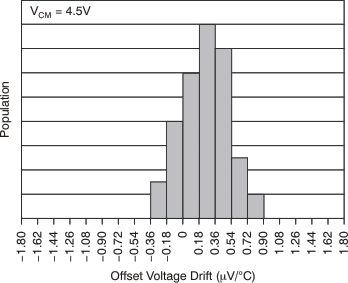Figure 8. Offset Voltage Drift (–40°C to 125°C)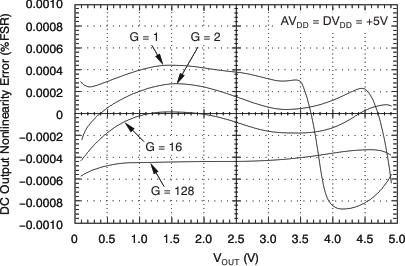Figure 10. PGA112 and PGA116 Nonlinearity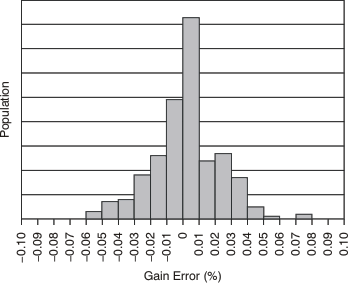Figure 12. Gain Error (1 < G ≤ 32)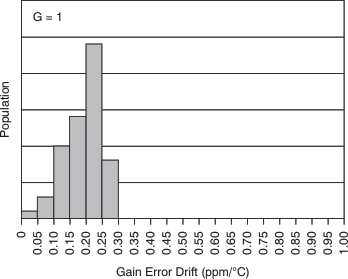Figure 14. Gain Error Drift (–40°C to 125°C)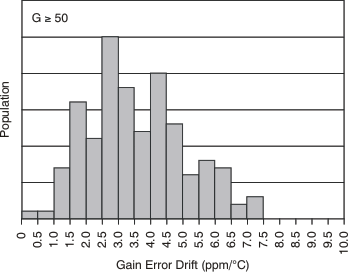Figure 16. Gain Error Drift (–40°C to 125°C)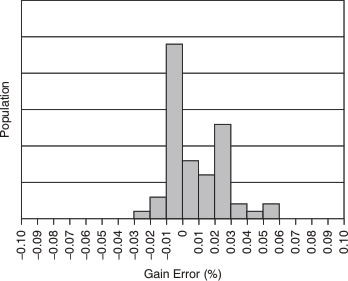Figure 18. CAL3 Gain Error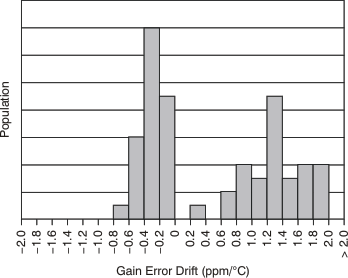Figure 20. CAL3 Gain Error Drift (–40°C to 125°C)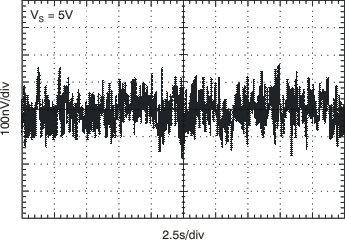Figure 22. 0.1 Hz to 10 Hz NOISE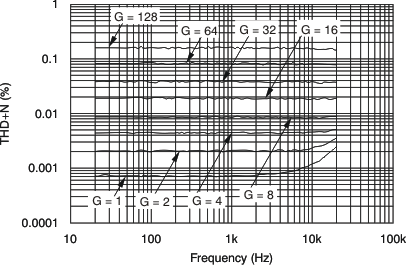Figure 24. PGA112, PGA116 THD + Noise vs Frequency (VOUT = 2 VPP)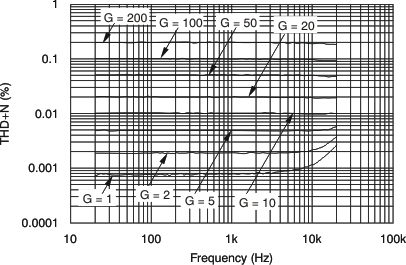Figure 26. PGA113, PGA117 THD + Noise vs Frequency (VOUT = 2 VPP)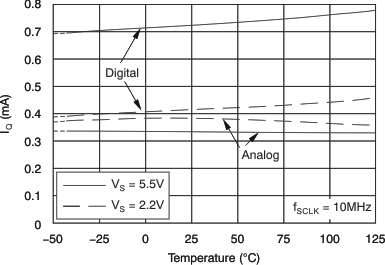Figure 28. Quiescent Current vs Temperature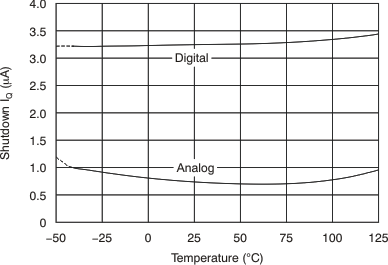Figure 30. Shutdown Quiescent Current vs Temperature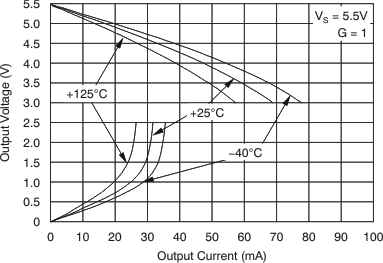Figure 32. Output Voltage vs Output Current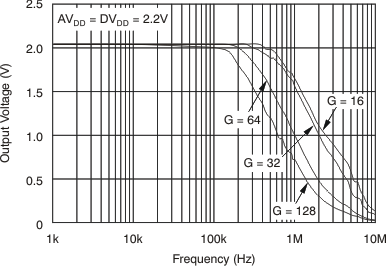Figure 34. PGA112, PGA116 Output Voltage Swing vs Frequency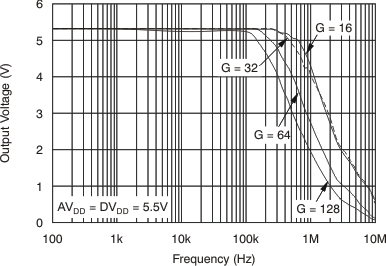Figure 36. PGA112, PGA116 Output Voltage Swing vs Frequency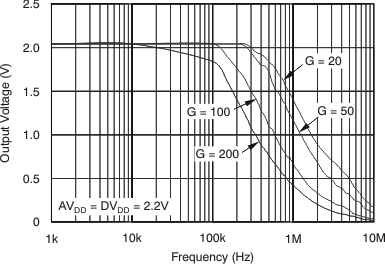Figure 38. PGA113, PGA117 Output Voltage Swing vs Frequency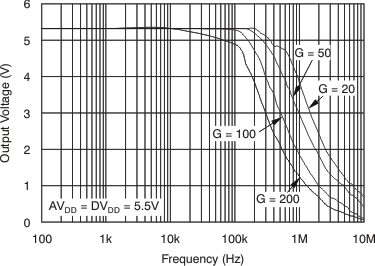Figure 40. PGA113, PGA117 Output Voltage Swing vs Frequency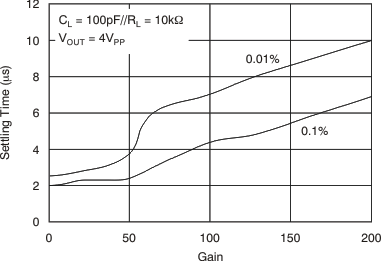Figure 42. Gain vs Settling Time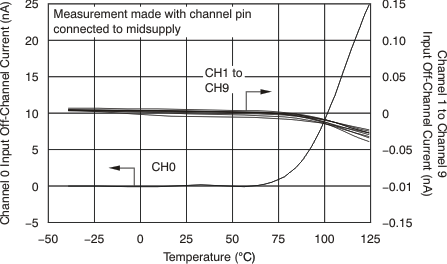Figure 44. Input OFF-Channel Leakage Current vs Temperature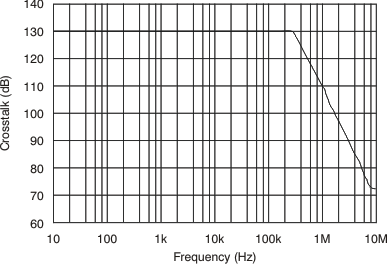Figure 46. Crosstalk vs FrequencyFigure 48. Small-Signal Pulse Response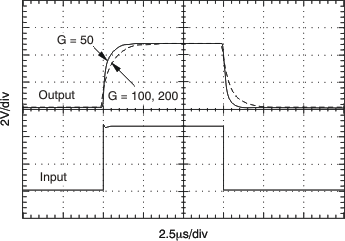Figure 50. Large-Signal Pulse Response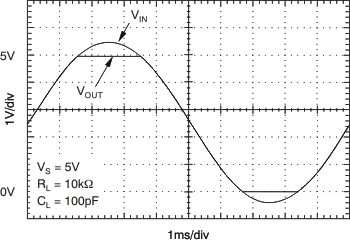Figure 52. Output Overdrive Performance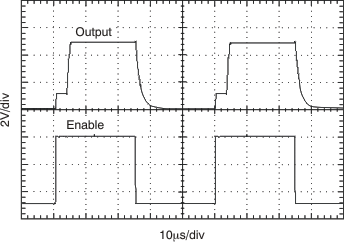Figure 54. PGA116, PGA117 Hardware Shutdown Mode